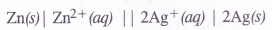## NCERT Solutions for Class 11 Chemistry Chapter 8 Redox Reactions

These Solutions are part of NCERT Solutions for Class 11 Chemistry. Here we have given NCERT Solutions for Class 11 Chemistry Chapter 8 Redox Reactions.

Question 1.
Assign oxidation numbers to the underlined elements in each of the following species ;
(a) NaH2PO4
(b) NaHSO4
(c) H4P2O7
(d) K2MnO4
(e) CaO2
(f) NaBH4
(g) H2S2O7
(h) KAl(SO4)2.12H2O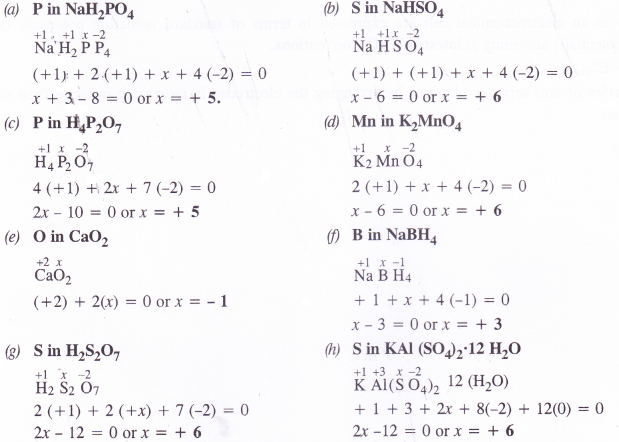Question 2.
What are the oxidation numbers of the underlined elements in each of the following and how do you rationalise your results ?|
(a)
KI(b) H2S4Oe (c) Fe3O4 (d) CH3CH2OH (e) CH3COOH         (H P. Board 2015)
(a) O.N. of iodine in KI3 : By conventional method, the oxidation number of iodine in the I3_ ion may be expressed as :Explanation : The oxidation number of iodine (I) comes out to be fractional which does not seem to be possible. Let us consider the structure of I3~ ion. In it, two atoms of iodine are linked to each other by covalent bond (I—I). The iodide ion (I~) is linked to the molecule by co-ordinate bond [I—I <—I]“. The molecule may be represented as K+[I—I <—1| . Now, in the anion, the oxidation number of the two I atoms is zero while I ion has – 1 oxidation number. The overall oxidation number of L“ ion is :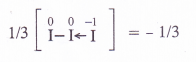(b) O.N. of S in H2S4O6 : By conventional method, the oxidation number of sulphur may be calculated as :
H+12  SxO-26
2 + 4x + 6 (- 2) =0 or 4x = 12 – 2 = 10 or x = 5/2
Explanation : In order to account for the fractional value, let us assign oxidation numbers to different sulphur atoms in the structural formula of the acid. The oxidation numbers of the two middle sulphur atoms is zero while the two atoms at the terminal positions have + 5 oxidation number.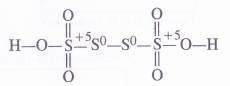Average O.N. of S = —1/4 [5+ 0 + 0 +5] = 5/2

(c) O. N. of Fe in Fe3O: By conventional method, oxidation number of Fe may be calculated as :
Fe3x 3 034
3x + 4(- 2) =0 or x = 8/3
Explanation : Fe3O4 is a mixed oxide and is an equimolar mixture of Fe+2 O-2 and Fe+32 O-23
Average O.N. of Fe = 1/3(2 + 2 x 3) =8/3

(d) O.N. of C in CH3CH2OH : By conventional method, the oxidation number of carbon in ethanol molecule may be calculated as :
CH3cH2OH or Cx2 H+16 O-2
2x + 6(+ 1) + (- 2) = 0 or 2x + 6 – 2 = 0 or 2x = – 4 or x = – 2
Explanation : Let us calculate the oxidation number of both C1 and C2 atoms.
C2 : The carbon atom is attached to three H atoms (less electronegative than carbon) and one CH2OH group (more electronegative than carbon).
∴ O.N. of C2 = 3(+ 1) +x + (- 1) = O or x = -2
C1 : The carbon atom is attached to one OH group (O.N. = – 1), two H atoms (O.N. = + 1) and one CH3 group (O.N. = + 1)
O.N. of C1 = ( + 1) + x + 2 (+ 1) + 1(- 1) =0 or x = – 2 Average O.N. of C = l/2[ – 2 + (- 2)] = – 2

(e) O.N. of C in CH3COOH : By conventional method the O.N. of carbon may be calculated as:
CH3COOH or c2 H4 62
2x + 4(+ 1) + 2(— 2) = 0 or x — 0
Explanation : Let us calculate the oxidation number of both C2 and C1 atoms.
C1 : The carbon atom is attached to three H atoms (less electronegative than carbon) and one COOH group (more electronegative than carbon).
∴ O.N. of C2 = 3 (+ 1) + x + 1(- 1) = 0 or x = – 2
C2 : The carbon atom is attached to one OH group (O.N. = – 1) one oxygen atom by double bond
(O.N. = – 2) and one CH3 group (O.N. = + 1)
O.N. of C1 = 1( + 1) + Jt + 1 (-2) + 1(- 1) = O or x = + 2
∴ Average O.N. of C = 1/2 [+ 2 + (- 2)] = 0

Question 3.
How will you justify that the following reactions are redox reactions in nature ?
(a)  CuO(s) + H2(g)  → Cu(s) + H2O(g)
(b) Fe2O3(s) + 3CO(g) →2Fe(s) + 3CO2(g)
(c) 2K(s) + F2(g)→2K+F(s)
(d) 4NH3(g) + 5O2(g) → 4NO(g) + 6H2O(g)
A chemical reaction may be regarded as redox reaction if one of the reacting species undergoes increase in O.N. (oxidation) and the other decrease in O.N. (reduction). Based upon this, let us try to justify that the reactions under study are redox reactions in nature.Question 4.
Fluorine reacts with ice as follows :
H2O(s) + F2(g) — HF(g) + HOF(g)
Justify that this reaction is a redox reaction.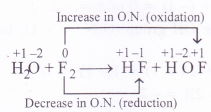In this reaction, the F2 molecule has undergone increase in O.N. in changing to HOF and decrease in O.N. in change to HF. It is therefore, a redox reaction. Since the same reacting species has undergone increase as well as decrease in O.N., it is also called disproportionation reaction.

Question 5.
Calculate the O.N. of sulphur, chromium and nitrogen in H2SO5, CrO5 and NO3 ion. Suggest structure of these compounds. Account for the fallacy if any.
(1) Oxidation number of S in H2SO5
By conventional method, O.N. of sulphur may be calculated as :
2 x 1 + x + 5 X (-2)= 0 or 2 + x – 10 = 0 or x = + 8 (wrong)
But this cannot be true as maximum oxidation number for sulphur cannot exceed +6 as it has only six valence electrons. The oxidation number of the element can be calculated from the structure of the persulphuric acid.
The sulphur atom is linked one OH group (O.N. = – 1) two oxygen atoms (O.N. = – 2) and one peroxide (- O – O -) linkage (O.N. = – 1)
O.N. of S = 1 (- 1) + x + 2(- 2) + 1( – 1) = 0
or
x = + 6

(2) Oxidation number of Cr in CrO5
By conventional method, O.N. of chromium may be calculated as :
x + 5 (-2) = 0 or x = + 10 (wrong)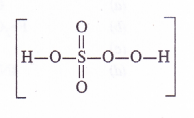This is wrong because maximum oxidation number of Cr cannot be more than +6, since it has only six electrons (3d54.s1) to take part in bond formation. The oxidation number of the metal atom can be calculated by taking into account its structure.
The Cr atom is linked with one oxygen atom by double bond (O. N. = – 2) and four oxygen atoms by peroxide linkages (O.N. = -1)
.’. O.N. of Cr = 4(- 1) \x + 1(- 2) = 0 or x = + 6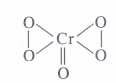(3) Oxidation number N in NO3 ion
By conventional method, O.N. of N atom in the ion may be calculated as : x + 3 (- 2) = – 1 or x = +5.This can be further made clear from the structure of the ion. In it, the nitrogen atom is linked to one single bonded oxygen atom (O.N. = – 2), one double bonded oxygen atom
(O.N.= – 2) and one oxygen atom by dative bond (O.N. = – 2).
O.N. of N = 1 (- 2) + x + 1( – 2) + 1 (- 2) = – 1 or x = + 5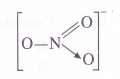Question 6.
Write the formulas of the following compounds :
(a) Mercury (II) chloride (b) Nickel (II) sulphate (c) Tin (IV) oxide (d) Thallium (I) sulphate (e) Iron (III) sulphate (f) Chromium (III) oxide.
The formulas have been shown by stock notations. The Roman numerals given in parenthesis represent the oxidation state of the metal atom. Taking into account the oxidation state of the anions, the chemical formulas of the compounds may be written as :
(a) HgCl2 (b) NiSO4 (c) SnO2 (d) T12SO4 (e) Fe2(SO4)3 (f) Cr2O3

Question 7.
Suggest a list of substances where carbon can exhibit oxidation states from -4 to +4 and nitrogen from -3 to +5.
Variable oxidation states of carbon :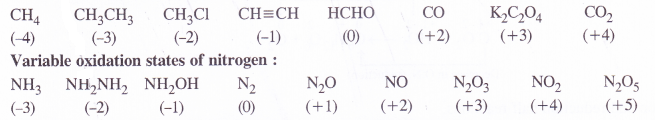Question 8.
While sulphur dioxide and hydrogen peroxide can act as oxidising as well as reducing agents in their reactions, ozone and nitric acid can act only as oxidising agents. Why ?
In sulphur dioxide (SO2) and hydrogen peroxide (H2O2), the oxidation states of sulphur and oxygen are +4 and -1 respectively. Since they can increase as well as decrease when these compounds take part in chemical reactions, they can act as oxidising as well as reducing agents. For example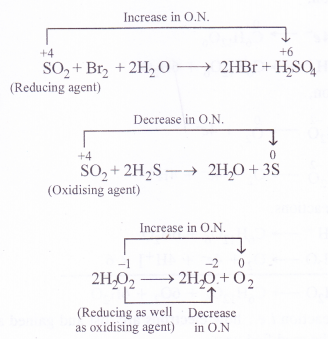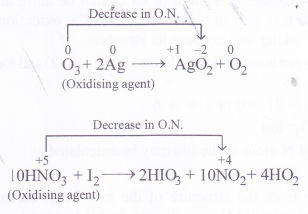In ozone (O3), the oxidation state of oxygen is zero while in nitric acid (HNO3), the oxidation state is nitrogen is +5. Since both them can undergo decrease in oxidation state and not an increase in its value, they can act only as oxidising agents and not as reducing agents.

Question 9.
Consider the reactions :
(a) 6CO 2(g) + 6H2O (l) → C6H12O6(s) + 6O2(g)
(b) O3(g) + H2O2(l) → H2O (l) + 2O2(g)
Why it is more appropriate to write these reactions as :
(a) 6CO2(g) + 12H2O(l)→ C6H12O6(s) + 6H2O(l) + 6O2(g)
(b) 63(g) + H2O2(l)→ H2O(l) + O2(g) + O2(g)
Also suggest a technique to investigate the path of the above (a) and (b) redox reactions.
(a)  6CO2(g) + 6H2O(l) → C6H6O6(s) + 6O2(g)
Let us try to balance the equation by ion-electron method.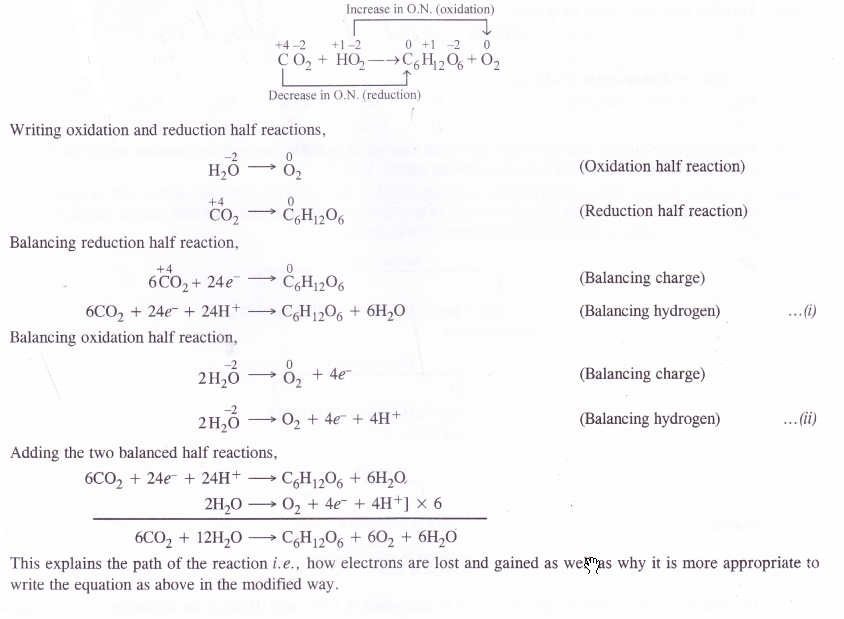Note:
(1) In this reaction O3 acts as an O.A. and H2O2 acts as a R.A.
(2) If a co-ordinate bond exist between two similar atoms, the donor atom acquires + 2 O.N. and the acceptor gets -2 O.N.
This explains the path of the reaction i.e., how electrons are lost and gained as well as and why it is more appropriate to write the equation as above.

Question 10.
The compound AgF2 is an unstable compound. However if formed, the compound acts as a very strong oxidising agent. Why ?
In AgFL the oxidation state of silver is + 2 i.e., it exists as Ag2+ ion. This is quite unstable since the normal stable oxidation state of the metal is + 1 or it exists as Ag+ ion. This means that if the compound AgF2 be formed, it will undergo reduction by the gain of electrons. It is therefore, a very strong oxidising agent
Ag2+ + e — Ag+

Question 11.
Whenever a reaction between an oxidising agent and a reducing agent is carried out, a compound of lower oxidation state is formed if the reducing agent is in excess and a compound of higher oxidation state is formed if the oxidising agent is in excess. Justify this statement giving three illustrationsQuestion 12.
(a) Though alkaline potassium permanganate and acidic potassium permanganate both are used as oxidants, yet in the manufacture of benzoic acid from toluene, we use alcoholic potassium permanganate as an oxidant, why ? Write a balanced redox equation for the reaction.

(b)
When concentrated sulphuric acid is added to an inorganic mixture containing chloride, we get a colourless pungent smelling gas HC1. However, if mixture contains bromide, then red vapours of bromine are evolved. Why ?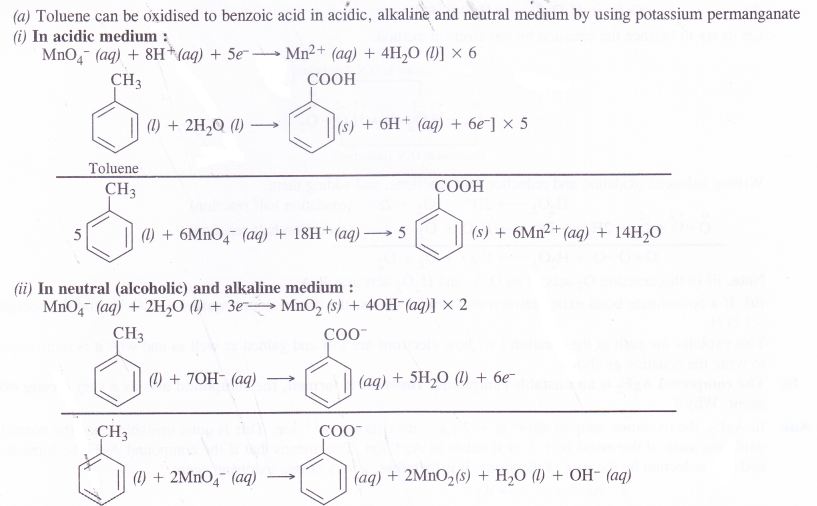In the manufacture of benzoic acid from toluene, neutral medium is preferred over acidic or alkaline medium due to following reasons.

•  In the neutral medium, neither acid or base is to be added externally. This will definitely reduce the cost of the manufacturing process.
• Alcohol if used as solvent will help in the formation of a homogeneous mixture between toluene (non-polar) and KMnO4 (ionic). Actually alcohol has non-polar alkyl group as well as polar OH group.

(b)
A chloride such as NaCl reacts with concentrated sulphuric acid to evolve hydrogen chloride gas upon heating. It has a pungent smell.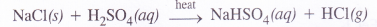Hydrogen bromide is also expected to be formed in the same way when a bromide is heated with concentrated sulphuric acid. However, the acid being a strong oxidising agent will oxidise HBr to bromine (Br2) which gets evolved  as red vapours.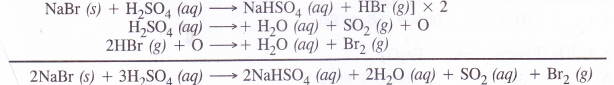Please note HC1 (g) is quite stable and is not oxidised to chlorine with concentrated sulphuric acid.

Question 13.
Identify the substance oxidised, reduced, oxidising agent and reducing agent for each of the following reactions :

1. 2AgBr(s) + C6H6O2(aq) →2Ag(s) + 2HBr(aq) + C6H4O2(aq)
2. HCHO(l) + 2[Ag(NH3)2]+(aq) + 3OH(aq) → 2Ag(s) + HCOO (aq) + 4NH3(aq) + 2H2O(l)
3. HCHO(l) + 2Cu2+(aq) + 5OH(aq)→ Cu2(O(s) + HCOO-(aq) + 3H2O(l)
4. N2H4(l) + 2H2O2(l) →N2(g) + 4H2O(l)
5. Pb(s) + PbO2(s) + 2H2SO4(aq)→ 2PbSO4(s) + 2H2O(l)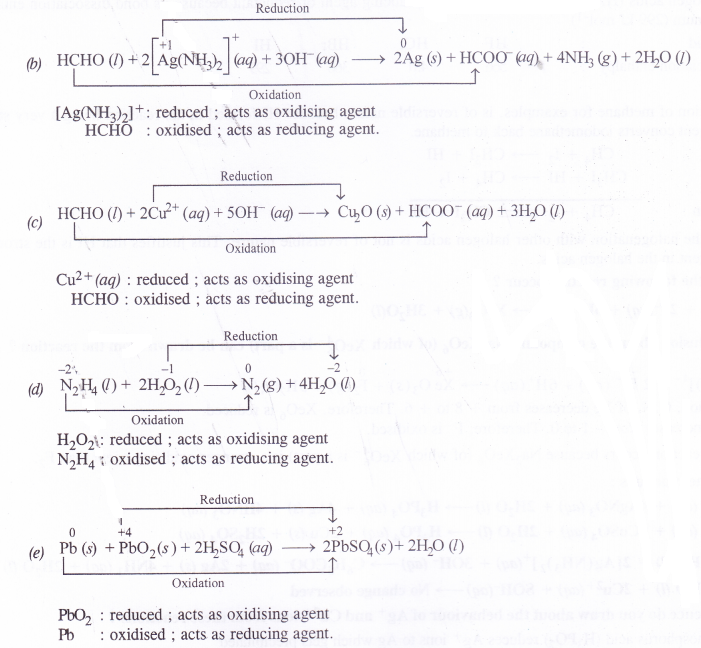AgBr         : reduced ; acts as oxidising agent
C6H6O2    : oxidised ; acts as reducing agent.

Question 14.
Consider the reactions :
2S2O2-3(aq) + I2(s) → S4O2-6(aq) + 2I(aq)
S2O(aq) + 2Br2(l) + 5H2O (l) → 2SO2-4(aq) + 4Br-(ag) + 10H +(aq)
Why does the same reductant the osulphate react differently with iodine and bromine ?
I2 oxidises thiosulphate ion to tetrathionate ion i.e., from O.S. of + 2 for S (in S2O2-3 ) to S.O. of + 5/2 for S (in S4O2-4 ion).
Br2 oxidises thiosulphate ion to sulphate ion i.e., from O.S. of + 2 for S (in S2O2-3) to O.S. of + 6 for S
(in SO2-4 ion)
This is because Br2 is a stronger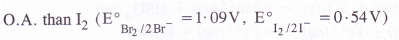Question 15.
Justify giving reactions that among halogens, fluorine is the best oxidant and among hydrohalic compounds, hydroiodic acid is the best reductant.
The halogens (X2) have strong electron accepting tendency. They are therefore, powerful oxidising agents. The relative order of oxidising powers of halogens is :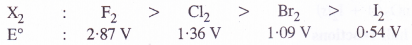Fluorine is the strongest oxidising agent (oxidant). It can be justified by the fact that it can liberate the other halogens from their respective compounds. For example,
2KC1 + F2  →  2KF + Cl2
2KBr + F2 → 2KF + Br2
2KI + F2 → 2KF + I2
Among halogen acids (HX), the HI is the strongest reducing agent or reductant because its bond dissociation enthalpy is the minimum (299 kJ mol-1)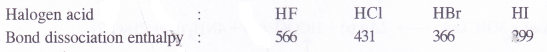(kJ mol-1)
The iodination of methane for examples, is of reversible nature because HI formed in the reaction being a very strong reducing agent converts iodomethane back to methaneHowever, the halogenation with other halogen acids is not of reversible nature. This justifies that HI is the strongest reducing agent in the halogen acids.

Question 16.
Why does the following reaction occur ?
XeO4-6 (aq) + 2F(aq) + 6H+(aq)→ XeO3(g) + 3H2O(l)
What conclusion about the compound Na4XeO6 (of which XeO4-6 is a part can be drawn from the reaction ?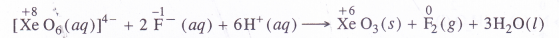In the reaction, O.N. of Xe decreases from + 8 to + 6. Therefore, XeO6 is reduced.O.N. of F increases from – 1 to 0. Therefore, F is oxidised. The redox reaction occurs because Na2XeO6 (of which XeOg“ is a part) is a stronger oxidising agent than F2.

Question 17.
Consider the reactions :
(a) H3PO2 (aq) + 4AgNO3 (aq) + 2H2O (l)→H3PO4 (aq) + 4Ag (s) + 4HNO3 (aq)
(b) H3PO2 (aq) + 2CuSQ4 (aq) + 2H2O (l)→ H3PO4 (aq) + 2Cu (s) + 2H2SO4 (aq)
(c) C6H5CHO (I) + 2[Ag(NH3)2+ (aq) + 3OH (aq)→ C6H5COO (aq) + 2Ag (s) + 4NH3 (aq) + 2H2O (l)
(d) C6H5CHO (l) + 2Cu2+(aq) + SOH(aq)→No change observed
What inference do you draw about the behaviour of Ag+ and Cu2+ ions from these reactions ?
(a) Hypophosphorus acid (H3PO2) reduces Ag+ ions to Ag which gets precipitated
(b) Hypophosphorus acid (H3PO2) reduces Cu2+ ions to Cu which gets precipitated
(C) Benzaldehyde (C6H5CHO) reduces Ag+ions to Ag which gets precipitated
(d) Benzaldehyde (C6H5CHO) is not in a position to reduce Cu2+ ions to Cu.
This shows that Ag+ ions are stronger oxidising agent than Cu2+ ions in all the reactions. This is quite evident from their relative positions in the reactivity series.

Question 18.
Balance the following equations by ion-electron method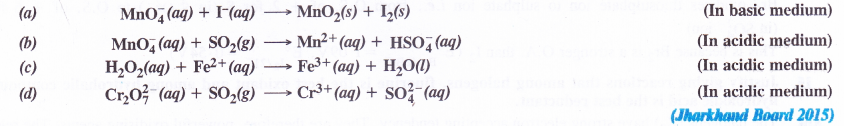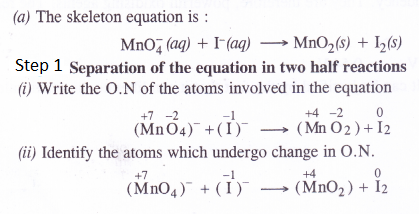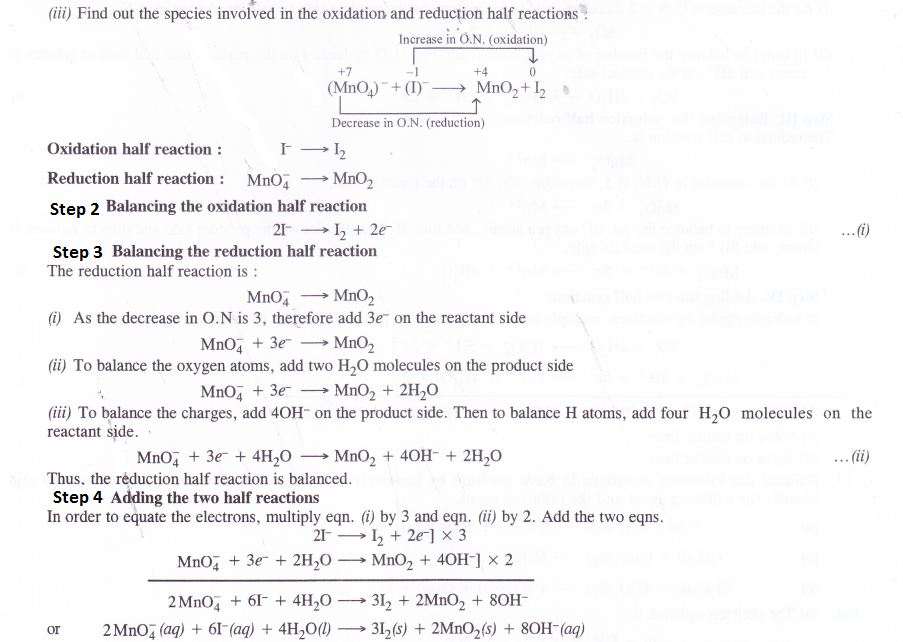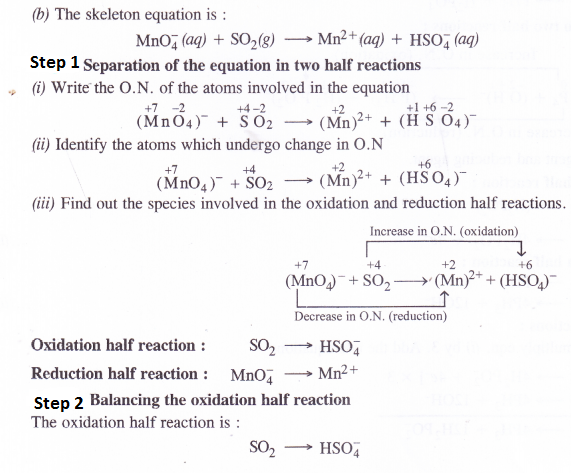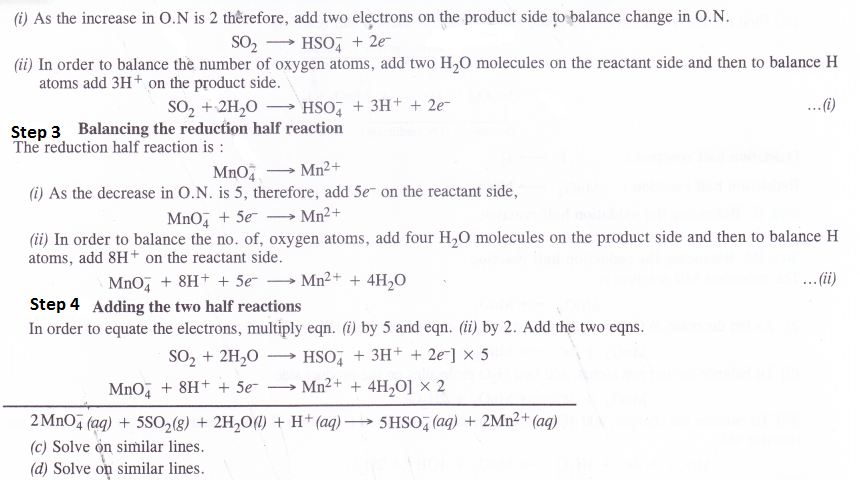Question 19.
Balance the following equations in basic medium by ion-electron method and oxidation number method and identify the oxidising agent and the reducing agent.
(a) p4(s) + OH+(aq) —> PH3(g) + H2PO2 (aq)
(b) N2H4(7) + CIO3 (aq) —> NOfe) + C l(g)
(c) C12O7(g) + H2O2(aq) —> CIO2(aq) + O2(g) + H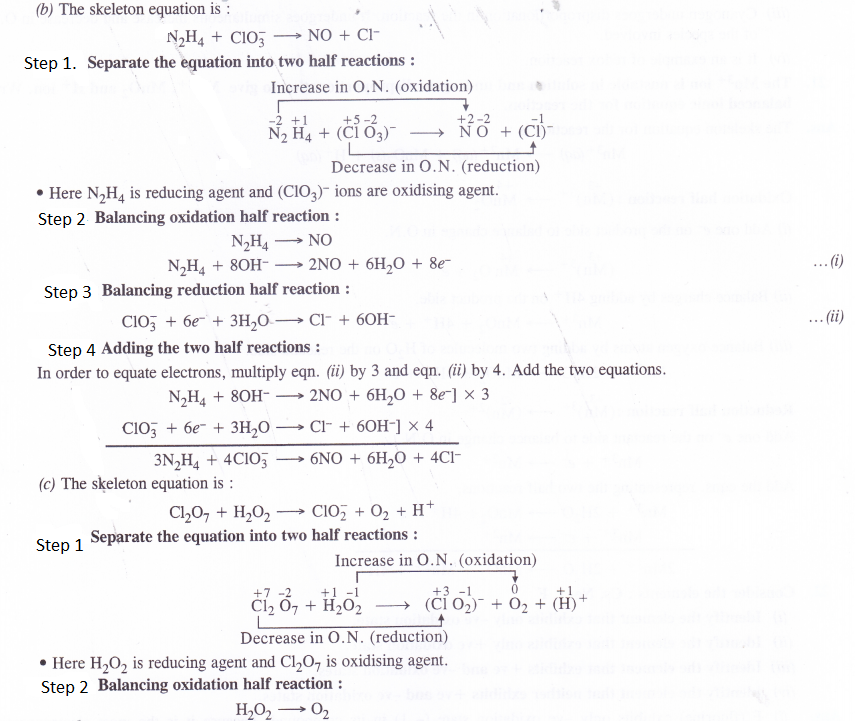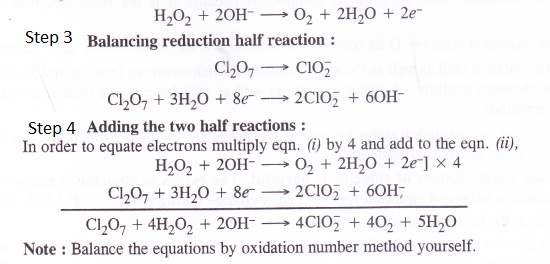Question 20.
Write four informations about the reaction :
(CN)2 (g) + 2OH (aq)—> CN (aq) + CNO (aq) + H2O (l).

1. The reaction involves the decomposition of cyanogen (CN)2 in the alkaline medium.
2. Both (CN)2 i.e. cyanogen and CN i.e. cyanide ions are pseudo halogens in nature i.e. they behave like halogens in characteristics.
3. Cyanogen undergoes disproportionation in the reaction. It undergoes simultaneous increase and decrease in O.N.of the species involved.
4. It is an example of redox reaction.

Question 21.
The Mn3+ ion is unstable in solution and under goes disproportionation to give Mn2+, MnO2 and H+ ion. Write balanced ionic equation for the reaction.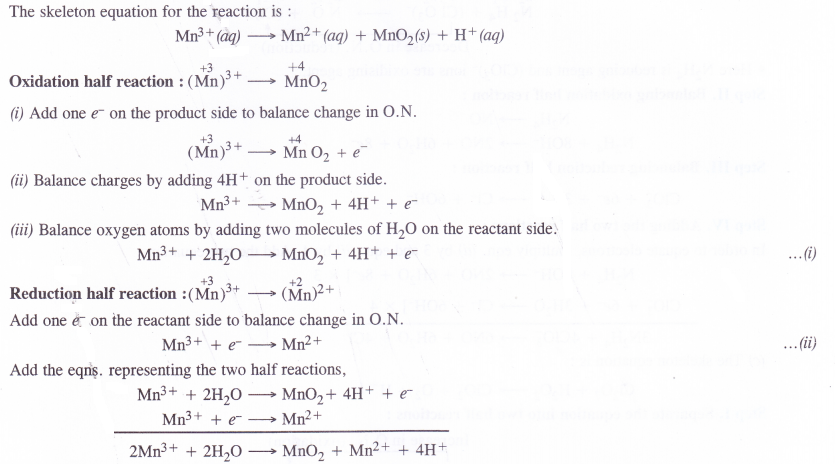Question 22.
Consider the elements : Cs, Ne, I, F

1. Identify the element that exhibits only -ve oxidation state.
2. Identify the element that exhibits only +ve oxidation state.
3. Identify the element that exhibits +ve and -ve oxidation states.
4. Identify the element that neither exhibits + ve and -ve oxidation states.

1. F (fluorine) exhibits only -ve oxidation state (- 1) in its compounds because it is the most electronegative element.
2. Cs (cesium) exhibits only +ve oxidation state (+ 1) its compounds because it is the most electropositive element.
3. Iodine has seven electrons in the valance shell as well as vacant 5d orbital to which electrons from 5p and 5s orbitals can be shifted. Therefore, the elements exhibits – 1 oxidation state as well as variable positive oxidation states of + 1, +3, +5 and +7 in its compounds.
4. Ne (neon) neither exhibits +ve nor -ve oxidation states because it is a noble gas element with completely filled orbitals (Is2 2s22p6). Moreover, it has no vacant d-orbitals.

Question 23.
Chlorine is used to purify drinking water. Excess of chlorine is harmful. The excess of chlorine is removed by treating with sulphur dioxide. Present a balanced equation for this redox change taking place.
(P.I.S.A. Based)
Chlorine reacts with sulphur dioxide in the presence of water as follows
Cl2 (aq) + SO2 (aq) + H2O (l) → Cl (aq) + SO42- (aq)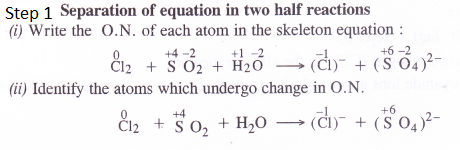Question 24.
From the periodic table, select three non-metals and three metals which can show disproportionation reactions.
Non-metals and metals which show variable oxidation states can take part in the disproportionation reactions. For examples,
Non-metals : The non-metals showing disproportionation reactions states are : P4, Cl2 and S8.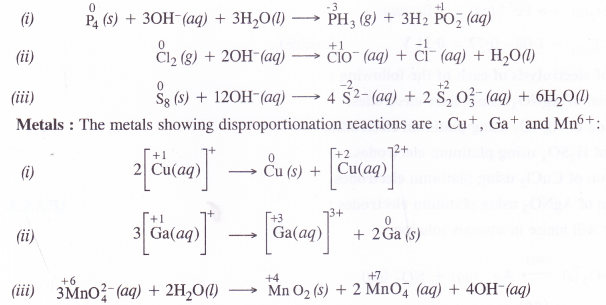Question 25.
In the Ostwald process for the manufacture of nitric acid, the first step involves the oxidation of ammonia gas by oxygen gas to give nitric oxide and steam. What is the maximum weight of nitric oxide that can be obtained starting only with 10.0 g of ammonia and reacting with 20.0 g of oxygen ?Question 26.

Using the standard electrode potentials given in the table, predict if the reaction between the following is feasible.
(a) Fe3+(aq) and I(aq)
(b) Ag+(aq) and Cu(s)
(c) Fe3+(aq) and Cu(s)
(d) Ag(s) and Fc3+(aq)
(f) Br2(aq) and Fe2+(aq).
A particular reaction can be feasible if e.m.f. of the cell based on the E° values is positive. Keeping this in mind, let us predict the feasibility of the reactions.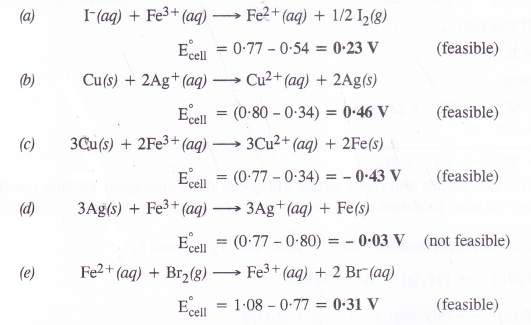Question 27.
Predict the products of electrolysis of each of the following :

1. An aqueous solution of AgNO3 using silver electrodes.
2. An aqueous solution of AgNO3 using platinum electrodes.
3. A dilute solution of H2SO4 using platinum electrodes.
4. An aqueous solution of CuCl2 using platinum electrodes.

(1) An aqueous solution of AgNO3 using platinum electrodes :     (P.I.S.A. Based)
Both AgNO3 and water will ionise in aqueous solutionAt cathode : Ag+ ions with less discharge potential are reduced in preference to H+ ions which will remain in solution. As a result, silver will be deposited at cathode.
Ag+ (aq) + e → Ag (deposited)
At anode : An equivalent amount of silver will be oxidised to Ag+ ions by releasing electrons.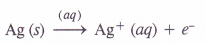As result of electrolysis, Ag from silver anode dissolves as Ag+(aq) ions while an equivalent amount of Ag+(aq) ions from the aqueous AgNO3 solution get deposited on the cathode.

(2) An aqueous solution of AgNO3 using platinum electrodes :

In the case, the platinum electrodes are the non-attackable electrodes. On passing current the following changes will occur at the electrodes.
At cathode : Ag+ ions will be reduced to Ag which will get deposited at the cathode.
At anode : Both NO3 and OH ions will migrate. But OH ions with less discharge potential will be oxidised in preference to NO3 ions which will remain in solution.
OH- (aq) → OH + e; 4OH → 2H2O(l) + O2 (g)
Thus, as a result of electrolysis, silver is deposited on the cathode while O2 is evolved at the anode. The solution will be acidic due to the presence of HNO3.

(3) A dilute solution of H2SO4 using platinum electrodes :
On passing current, both acid and water will ionise as follows :At cathode :
H+ (aq) ions will migrate to the cathode and will be reduced to H2.
H+ (aq) + e-→ H ; H + H→ H2 (g)
Thus, H2 (g) will evolve at cathode.
At anode : OH ions will be released in preference to SO2-ions because their discharge potential is less. They will be oxidised as follows :
OH (aq) → OH + e ; 4OH → 2H2O(l) + O2 (g)
Thus, O2 (g) will be evolved at anode. The solution will be acidic and will contain H2SO4.

(4) An aqueous solution of CuCl2 using platinum electrodes :

The electrolysis proceeds in the same manner as discussed in the case of AgNO3 solution. Both CuCl2 and H2O will ionise as follows :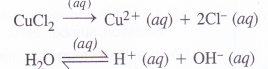At cathode :  Cu2+ ions will be reduced in preference to H+ ions and copper will be deposited at cathode.
Cu2+ (aq) + 2e → Cu (deposited)
At anode : Cl ions will be discharged in preference to OH ions which will remain in solution.
Cl→Cl+ e ; Cl + Cl → Cl2 (g) (evolved)
Thus, Cl2 will evolve at anode.

Question 28.
Arrange the following metals in the order in which they displace each other from their salts. Al, Cu, Fe, Mg and Zn
This is based upon the relative positions of these metals in the activity series. The metal placed lower in the series can displace the metals occupying a higher position present as its salt. Based upon this, the correct order is : Mg, Al, Zn, Fe, Cu.

Question 29.
Given the standard electrode potentials
K+/K = – 2.93 V, Ag+/Ag = 0.80V
Hg2+/Hg = 0.79 V ; Mg2+/Mg = – 2.37 V, Cr3+/Cr = – 0.74 Y
Arrange these metals in increasing order of their reducing power.
If may be noted that lesser the E° value for an electrode, more will be reducing power. The increasing order of reducing power is :
Ag < Hg < Cr < Mg < K.

Question 30.
Depict the galvanic cell in which the reaction Zn(s) + 2Ag +(aq)→ Zn2+(aq) + 2Ag(s) takes place. Further show :
(1) which electrode is negatively charged.
(2) the carriers of the current in the cell.
(3) individual reaction at each electrode.